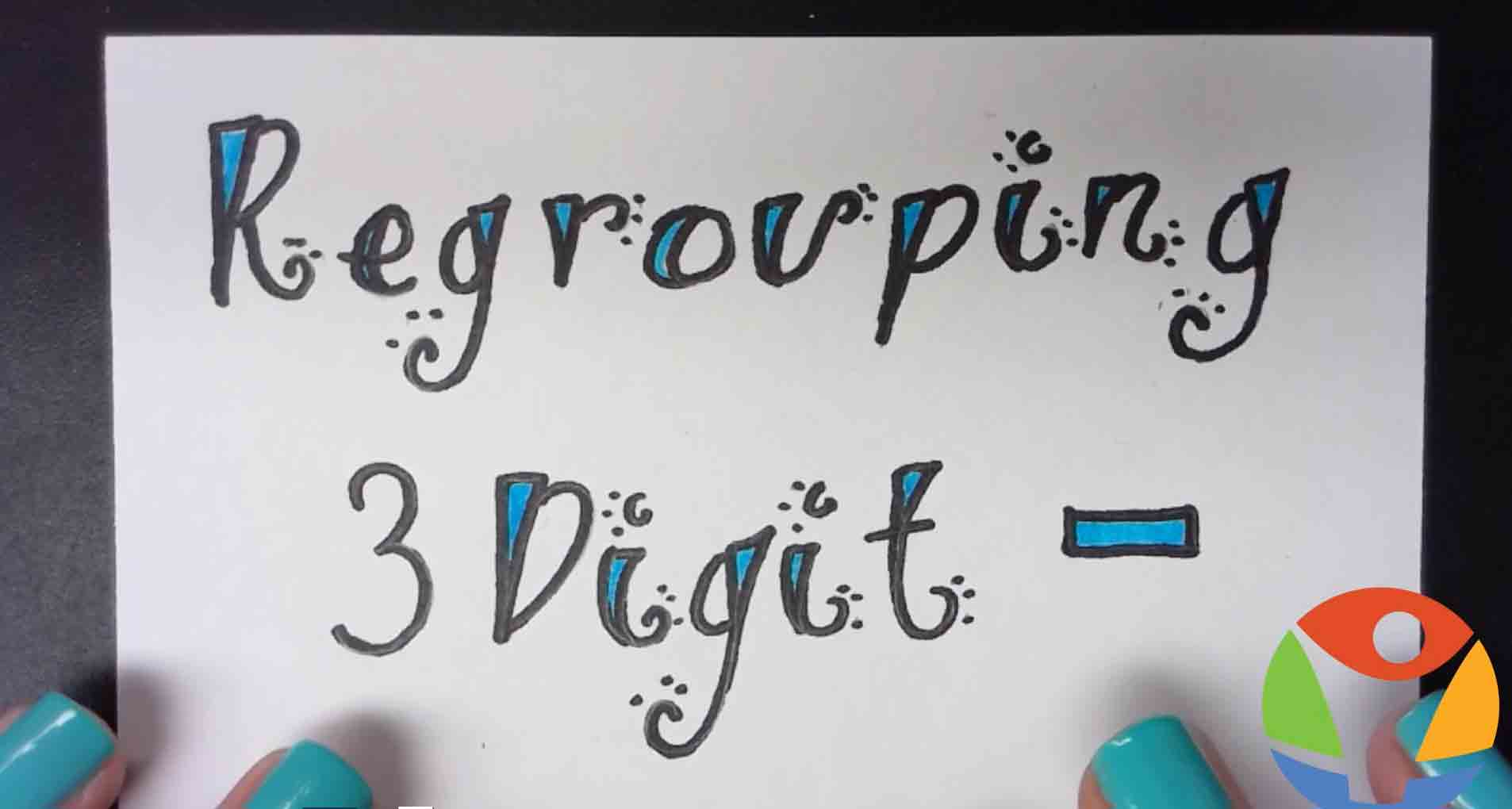Subtraction with Regrouping 3 Digits# Mastering Subtraction with Regrouping (3 digits)

## What is Regrouping?

Regrouping, commonly called borrowing or carrying over, is the process of forming groups of tens to solve addition or subtraction problems. Although regrouping can be used to solve both addition and subtraction problems, this section will focus on subtraction with regrouping of 3-digit numbers. Examples of subtraction-with-regrouping of three digit number are provided in the video below.

• A video on the subtraction of 3 digit numbers with regrouping.
• 3-digit subtraction with regrouping worksheets pdf for practice.
• Some additional resources on regrouping.
• A supplementary tool to solidify the regrouping concept.

## 3 Digit Subtraction with Regrouping Pdf Worksheets

An important part of the learning process is to practice what you've just learned to solidify the learned concepts from the video. To help with this, we have provided some free '3-digit subtraction with regrouping' worksheets, which you can access by clicking below.

## A 'Subtraction of 3 Digit Numbers with Regrouping' Resource

Thanks for visiting Sqooasha. If you need more worksheets than the free ones that have been provided, additional worksheets on addition and subtraction can be accessed on our worksheets page. You can also access additional, bundled free worksheets with solutions, which cover addition, subtraction, multiplication, division and randomized worksheets by clicking the "Free Worksheets" button below.

## Sqooasha Platform

If you do not feel like manually doing all the grading for your child, subscribe to a Sqooasha plan for the platform to automatically do the grading for you . Click below to start your free 7-day trial!

You may also be interested in our '3 digit Addition with Regrouping' article, and may want to check out our Subtraction without Regrouping video. We hope to see you again soon.